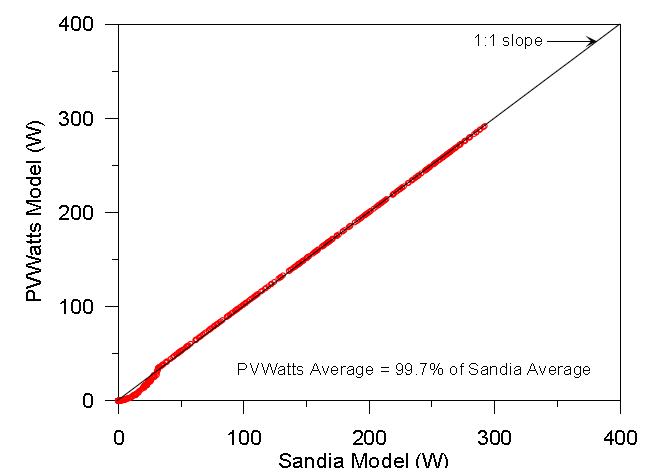# PVWatts

The PVWatts model is provided by the National Renewable Energy Laboratory (NREL) as an online PV performance modeling application.  The equations that underlie PVWatts are really quite simple.  There are two primary input variables:

• Effective irradiance ($$E_e$$)
• PV cell temperature ($$T_c$$)

PVWatts relates these two variables to the maximum power point ($$P_{mp}$$) with two equations depending on the irradiance level.

For POA irradiance > 125 W/$$m^{2}$$ :

$$P_{mp}=\frac{E_{e}}{E_{0}}P_{mp0}\left [ 1+\gamma \left ( T_{c}-T_{0} \right ) \right ]$$

where $$E_{0}$$ and $$T_{0}$$ are reference irradiance (1000 W/$$m^{2}$$ ) and reference temperature (25° C), respectively.  $$\gamma$$ is the temperature correction for maximum power ($$P_{mp}$$).

For POA irradiance <= 125 W/$$m^{2}$$ :

$$P_{mp}=\frac{0.008\left(E_{e} \right )^{2} }{E_{0}}P_{mp0}\left [ 1+\gamma \left ( T_{c}-T_{0} \right ) \right ]$$.

This modified output at low irradiance was observed by researchers at Sandia National Laboratories and introduced in a early model called PVFORM.

PVWatts is designed for x-Si modules and is not recommended for thin film technologies.  Below is an example from Marion (2010) comparing performance predictions from PVWatts and the Sandia PV Array Performance Model (SAPM).From Marion (2010) presentation: “Overview of the PV Module Model in PVWatts”Marion has proposed improvements to the PVWatts model which are described here.